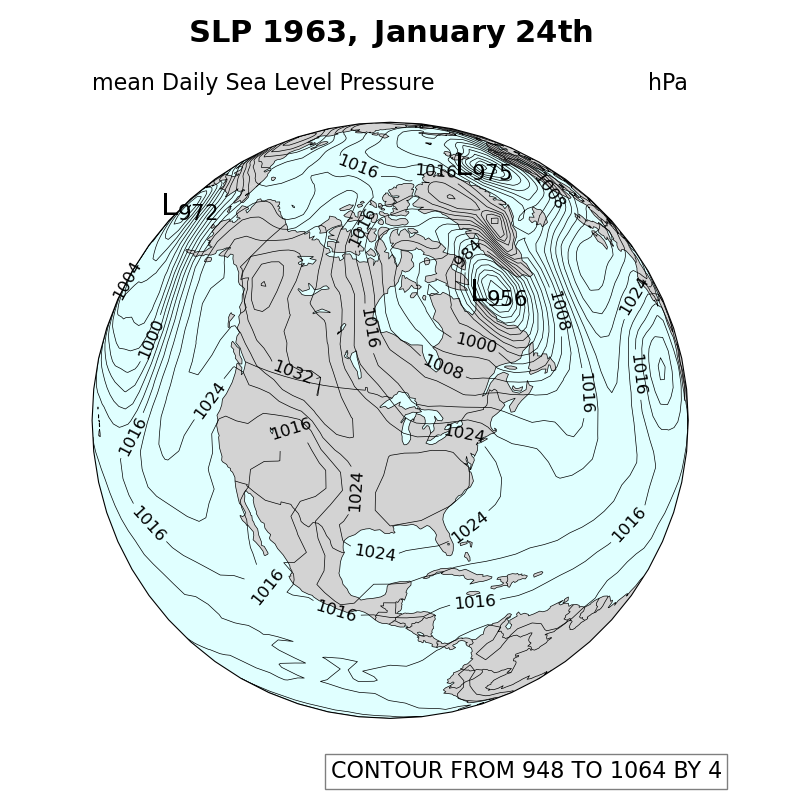# NCL_sat_1.py#

This script illustrates the following concepts:
• Creating an orthographic projection

• Drawing line contours over a satellite map

• Manually labeling contours

• Transforming coordinates

See following URLs to see the reproduced NCL plot & script:

Import packages:

import xarray as xr
import matplotlib.pyplot as plt
import cartopy.crs as ccrs
import cartopy.feature as cfeature
import numpy as np

import geocat.datafiles as gdf
import geocat.viz as gv


# Open a netCDF data file using xarray default engine and
# load the data into xarrays
ds = xr.open_dataset(gdf.get("netcdf_files/slp.1963.nc"), decode_times=False)

# Get data from the 24th timestep
pressure = ds.slp[24, :, :]

# Translate short values to float values
pressure = pressure.astype('float64')

# Convert Pa to hPa data
pressure = pressure * 0.01

# Fix the artifact of not-shown-data around 0 and 360-degree longitudes


Create plot

# Set figure size
fig = plt.figure(figsize=(8, 8))

# Set global axes with an orthographic projection
proj = ccrs.Orthographic(central_longitude=270, central_latitude=45)
ax = plt.axes(projection=proj)
ax.set_global()

# Add land, coastlines, and ocean features
facecolor='lightcyan',
edgecolor='black',
linewidth=.5)

# Make array of the contour levels that will be plotted
contours = np.arange(948, 1072, 4)

# Plot contour data
p = wrap_pressure.plot.contour(ax=ax,
transform=ccrs.PlateCarree(),
linewidths=0.5,
levels=contours,
cmap='black',

# regular pressure contour levels- These values were found by setting
# 'manual' argument in ax.clabel call to 'True' and then hovering mouse
# over desired location of countour label to find coordinate
# (which can be found in bottom left of figure window).
regularCLabels = [(176.4, 34.63), (-150.46, 42.44), (-142.16, 28.5),
(-134.12, 16.32), (-108.9, 17.08), (-98.17, 15.6),
(-108.73, 42.19), (-111.25, 49.66), (-127.83, 41.93),
(-92.49, 25.64), (-77.29, 29.08), (-77.04, 16.42),
(-95.93, 57.59), (-156.05, 84.47), (-17.83, 82.52),
(-76.3, 41.99), (-48.89, 41.45), (-33.43, 37.55),
(-46.98, 17.17), (1.79, 63.67), (-58.78, 67.05),
(-44.78, 53.68), (-69.69, 53.71), (-78.02, 52.22),
(-16.91, 44.33), (-95.72, 35.17), (-102.69, 73.62)]

# low pressure contour levels- these will be plotted
# as a subscript to an 'L' symbol.
lowCLabels = gv.findLocalExtrema(pressure,
eType='Low',
highVal=1040,
lowVal=975)

# Plot Clabels
gv.plotCLabels(ax, p, ccrs.Geodetic(), proj, clabel_locations=regularCLabels)
gv.plotELabels(pressure, ccrs.Geodetic(), proj, clabel_locations=lowCLabels)

# Use gv function to set title and subtitles
gv.set_titles_and_labels(ax,
maintitle=r"$\bf{SLP}$" + " " + r"$\bf{1963,}$" + " " +
r"$\bf{January}$" + " " + r"$\bf{24th}$",
maintitlefontsize=20,
lefttitle="mean Daily Sea Level Pressure",
lefttitlefontsize=16,
righttitle="hPa",
righttitlefontsize=16)

# Set characteristics of text box
props = dict(facecolor='white', edgecolor='black', alpha=0.5)

# Place text box
ax.text(0.40,
-0.1,
'CONTOUR FROM 948 TO 1064 BY 4',
transform=ax.transAxes,
fontsize=16,
bbox=props)

# Make layout tight
plt.tight_layout()

plt.show()Total running time of the script: ( 0 minutes 2.913 seconds)

Gallery generated by Sphinx-Gallery